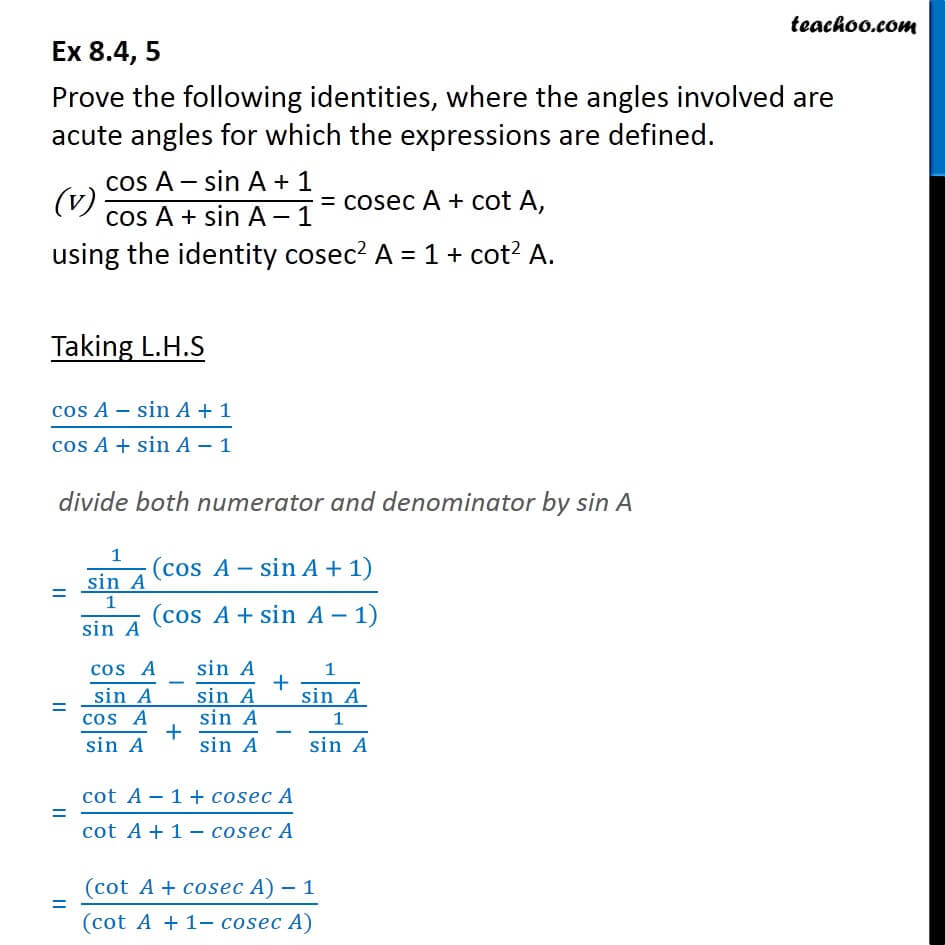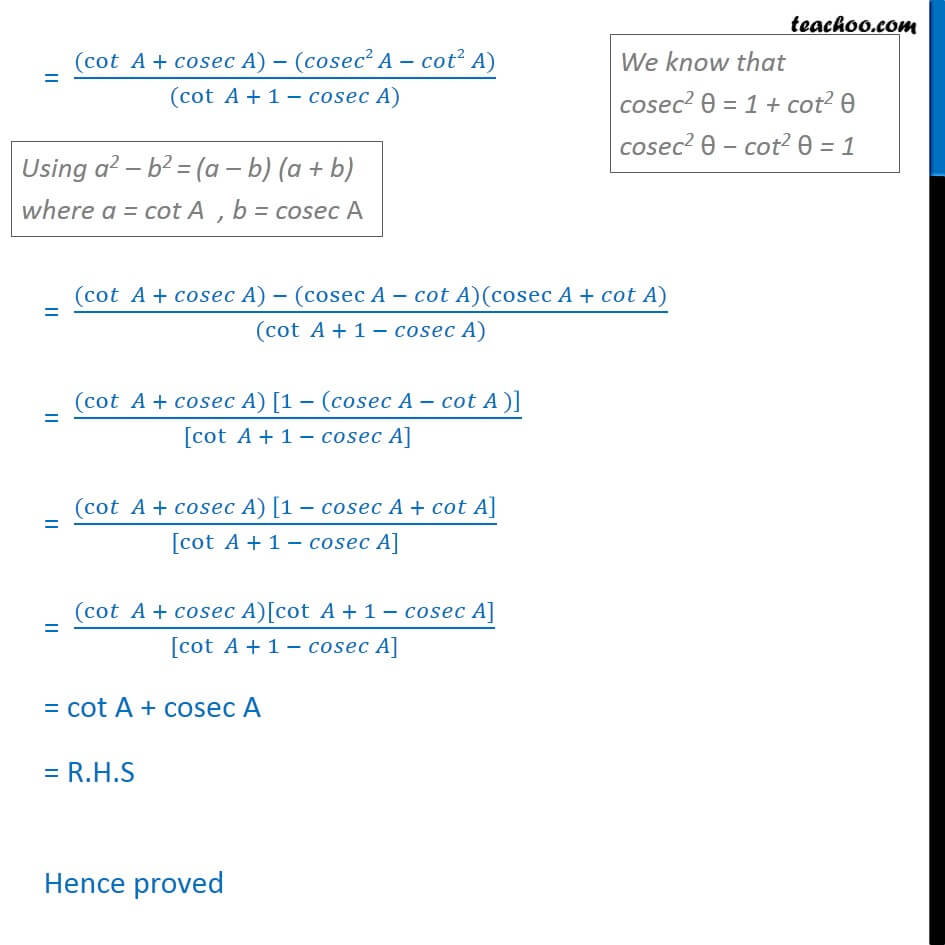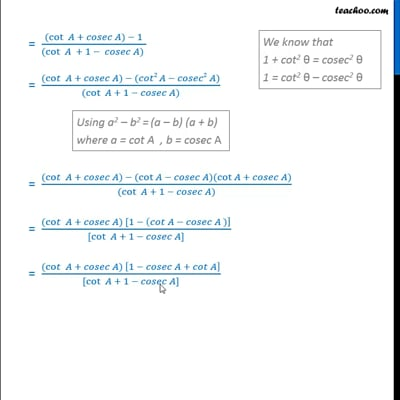Ex 8.4

Chapter 8 Class 10 Introduction to Trignometry
Serial order wiseThis video is only available for Teachoo black users

Get live Maths 1-on-1 Classs - Class 6 to 12

### Transcript

Ex 8.4, 5 Prove the following identities, where the angles involved are acute angles for which the expressions are defined. "cos A sin A + 1" /"cos A + sin A 1" = cosec A + cot A, using the identity cosec2 A = 1 + cot2 A. Taking L.H.S (cos sin + 1)/(cos + sin 1) divide both numerator and denominator by sin A = (1/sin (cos sin + 1 ))/(1/sin (cos + sin 1 ) ) = (cos /sin sin /sin + 1/sin )/(cos /sin + sin /sin 1/sin ) = cot 1 + /cot + 1 = ((cot + ) 1 )/((cot + 1 ) ) = ((co + ) ( 2 2 ) )/((cot + 1 )) = ((co + ) (cot )(cot + ) )/((cot + 1 )) = ((co + ) [1 ( )] )/([cot + 1 ]) = ((co + ) [1 + ] )/([cot + 1 ]) = cot A + cosec A = R.H.S Hence proved